StatLect

# Boosted classifier

We have already studied how gradient boosting and decision trees work, and how they are combined to produce extremely powerful predictive models, called boosted trees. However, until now we have applied boosting and decision trees only to regression problems.

Here we apply these techniques to a classification problem and we show that a boosted classifier built with the LightGBM algorithm significantly outperforms other classifiers.## Data set

We use the same artificially-generated data set used in a previous notebook, but the output is transformed to categorical (1 if the continuous output from the previously used data set is above its sample median, 0 otherwise):

• there are 300 correlated variables in the input vector;

• the outputis a function of only 10 of them;

• the 10 relevant inputs have:

• linear effects;

• non-linear effects (square, log, cos);

• interaction effects (products);

• threshold effects (some are relevant only if others are above threshold);

• there are 500 observations in the data set.

## Models

In our Python examples, we will show the performance of different classifiers:

• a plain vanilla logit model;

• a gradient-boosted logit in which the base learners are uni-variate linear regressions;

• a gradient-boosted logit in which the base learners are decision trees (built with LightGBM).

## Logit model

Our prediction ofis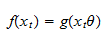where the inputis a row vector, the parameteris a vector of regression coefficients, and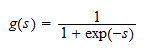is the logistic function.

The loss function we use is the log-loss:which can be minimized numerically using standard algorithms implemented in most statistical software packages.

### Import the data and use scikit-learn to split into train-val-test (60-20-20)

We first import the data and split it into training, validation and test.

``````# Import the packages used to load and manipulate the data
import numpy as np # Numpy is a Matlab-like package for array manipulation and linear algebra
import pandas as pd # Pandas is a data-analysis and table-manipulation tool

# Import the function that performs sample splits from scikit-learn
from sklearn.model_selection import train_test_split

try:
except:
y = y.values # Transform y into a numpy array
y = (y > np.median(y)) # Transform the output to categorical

# Print some information about the output variable
print('Class and dimension of output variable:')
print(type(y))
print(y.shape)

# Load the input variables with pandas
try:
except:
x = x.values

# Print some information about the input variables
print('Class and dimension of input variables:')
print(type(x))
print(x.shape)

# Create the training sample
x_train, x_val_test, y_train, y_val_test
= train_test_split(x, y, test_size=0.4, random_state=0)

# Split the remaining observations into validation and test
x_val, x_test, y_val, y_test
= train_test_split(x_val_test, y_val_test, test_size=0.5, random_state=0)

# Print the numerosities of the three samples
print('Numerosities of training, validation and test samples:')
print(x_train.shape, x_val.shape, x_test.shape)``````

The output is:

``````Class and dimension of output variable:
class 'numpy.ndarray'
(500, 1)
Class and dimension of input variables:
class 'numpy.ndarray'
(500, 300)
Numerosities of training, validation and test samples:
300 100 100``````

### Train the logit model

We use scikit-learn's LogisticRegression function to train our logit model.

Note that the predict method outputs a True value if the predicted probability is above 0.5 and a False value otherwise. The accuracy_score is the percentage of predictions that coincide with the actual value.

Also note that the validation set is never used in the training of the logit model. Therefore, we can use it as a second test set.

``````# Import packages and functions from scikit-learn
from sklearn import linear_model
from sklearn.metrics import log_loss, accuracy_score

# Create logit object
logit = linear_model.LogisticRegression(fit_intercept=True, max_iter=1000, penalty='none')

# Train the model using the training set
logit.fit(x_train, y_train)

# Make predictions on the training and validation sets
y_train_pred = logit.predict(x_train)
y_val_pred = logit.predict(x_val)
y_test_pred = logit.predict(x_test)

# Print empirical risk on all sets
print('Log-loss on training set:')
print(log_loss(y_train, y_train_pred))
print('Log-loss on validation set:')
print(log_loss(y_val, y_val_pred))
print('Log-loss on test set:')
print(log_loss(y_test, y_test_pred))
print('')

# Print accuracy on all sets
print('Accuracy on training set:')
print(accuracy_score(y_train, y_train_pred))
print('Accuracy on validation set:')
print(accuracy_score(y_val, y_val_pred))
print('Accuracy on test set:')
print(accuracy_score(y_test, y_test_pred))``````

The output is:

``````Log-loss on training set:
9.992007221626415e-16
Log-loss on validation set:
19.687326432379542
Log-loss on test set:
17.269612084735794

Accuracy on training set:
1.0
Accuracy on validation set:
0.43
Accuracy on test set:
0.5``````

Overfitting is so severe that the logit is able to make perfect predictions on the training set, but forecasts on the test are not more accurate than those made by flipping a coin.

## Gradient-boosted logit with linear base learners

We now train a gradient-boosted logit in which the base learners are uni-variate linear regressions.

As before, our prediction ofis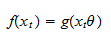where the inputis a row vector, the parameteris a column vector of regression coefficients, andis the logistic function.

The vector of regression coefficientswill be set iteratively, by gradient boosting.

The loss function we use is the log-loss:We start fromThen, at each iteration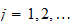, we perform the following steps:

1. we compute the pseudo-residuals from the previous iteration: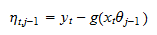2. we find the input variable that has the highest correlation (in absolute value) with the pseudo-residuals (on the training sample);

3. we estimate by ordinary least squares (on the training sample) the coefficientof the uni-variate regression of the residuals on the chosen variable (suppose it is the-th);

4. we set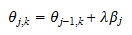whereis the learning rate (usually); a learning rate less than 1 is used so as to have a gradual increase in complexity and overfitting; all the other entries ofare left unchanged;

5. we compute the empirical risk (average log-loss) of the predictionson the validation sample;

6. if the empirical risk on the validation sample has not been decreasing for a pre-set number of iterations, we stop the algorithm.

The boosted logit, that we use to make predictions, is the most complex one, produced in the last iteration of the algorithm.

The Python code is obtained by slightly modifying the code previously used for boosted linear regressions. The changes are marked by comments to the code.

### Train the boosted logit

``````# Import package used to make copies of objects
from copy import deepcopy

# Our boosted logit (blogit) class will implement 3 methods
# (constructor, fit, and predict), as previously seen in scikit-learn
class blogit:
def __init__(self, learning_rate, max_iter, early_stopping):
self.lr = learning_rate
self.max_iter = max_iter
self.early = early_stopping
self.x_mean = 0
self.x_std = 1
self.theta = 0
self.mses = []

def fit(self, x_train_0, y_train_0, x_val_0, y_val_0):
# Make copies of data to avoid over-writing original dataset
x_train = deepcopy(x_train_0)
y_train = deepcopy(y_train_0)
x_val = deepcopy(x_val_0)
y_val = deepcopy(y_val_0)

# De-mean the input variables
self.x_mean = np.mean(x_train, axis=0, keepdims=True)
x_train -= self.x_mean
x_val -= self.x_mean

# Standardize the input variables
self.x_std = np.std(x_train, axis=0, keepdims=True)
x_train /= self.x_std
x_val /= self.x_std

# Initialize counters (total boosting iterations and unproductive iterations)
current_iter = 0
no_improvement = 0

# The starting model has all coefficients equal to zero and predicts that the two classes are equally likely
self.theta = np.zeros((x_train.shape, 1))
y_train_scores = 0 * y_train # Inputs to logistic function
y_train_pred = 0.001 + 0.998 / (1 + np.exp(- y_train_scores)) # Logistic transformation
y_val_scores = 0 * y_val  # Inputs to logistic function
y_val_pred = 0.001 + 0.998 / (1 + np.exp(- y_val_scores)) # Logistic transformation
eta = y_train - y_train_pred # Pseudo-residuals
log_losses = [np.mean(- y_val * np.log(y_val_pred) - (1 - y_val) * np.log(1 - y_val_pred))] # Log-loss

# Boosting iterations
while no_improvement < self.early and current_iter < self.max_iter:
current_iter += 1
corr_coeffs = np.mean(x_train * eta, axis=0)
index_best = np.argmax(np.abs(corr_coeffs))
self.theta[index_best] += self.lr * corr_coeffs[index_best]
y_train_scores += self.lr * corr_coeffs[index_best] * x_train[:, [index_best]] # Inputs to logistic function
y_train_pred = 0.001 + 0.998 / (1 + np.exp(- y_train_scores)) # Logistic transformation
eta = y_train - y_train_pred # Pseudo-residuals
y_val_scores += self.lr * corr_coeffs[index_best] * x_val[:, [index_best]] # Inputs to logistic function
y_val_pred = 0.001 + 0.998 / (1 + np.exp(- y_val_scores)) # Logistic transformation
log_losses.append(np.mean(- y_val * np.log(y_val_pred) - (1 - y_val) * np.log(1 - y_val_pred))) # Log-loss
if log_losses[-1] > np.min(log_losses[0:-1]):
no_improvement += 1
else:
no_improvement = 0

# Final output message
print('Boosting stopped after ' + str(current_iter) + ' iterations')

def predict(self, x_test_0):
# Make copies of the data to avoid over-writing original dataset
x_test = deepcopy(x_test_0)

# De-mean input variables using means on training sample
x_test = x_test - self.x_mean

# Standardize output variables using standard deviations on training sample
x_test = x_test / self.x_std

# Return prediction
y_test_scores = np.dot(x_test,self.theta)
return 0.001 + 0.998 / (1 + np.exp(- y_test_scores))

# Create a boosted logit object
bl = blogit(0.1, 10000, 20)

# Train the model
bl.fit(x_train, y_train.astype('float64'), x_val, y_val.astype('float64'))

# Make predictions on the train, validation and test sets
y_train_pred = bl.predict(x_train)
y_val_pred = bl.predict(x_val)
y_test_pred = bl.predict(x_test)

# Print empirical risk on all sets
print('Log-loss on training set:')
print(log_loss(y_train, y_train_pred))
print('Log-loss on validation set:')
print(log_loss(y_val, y_val_pred))
print('Log-loss on test set:')
print(log_loss(y_test, y_test_pred))
print('')

# Print Accuracy on all sets
print('Accuracy on training set:')
print(accuracy_score(y_train, y_train_pred > 0.5))
print('Accuracy on validation set:')
print(accuracy_score(y_val, y_val_pred > 0.5))
print('Accuracy on test set:')
print(accuracy_score(y_test, y_test_pred > 0.5))``````

The output is:

``````Boosting stopped after 20 iterations
Log-loss on training set:
0.682572790642433
Log-loss on validation set:
0.6947553369260098
Log-loss on test set:
0.6918729538918865

Accuracy on training set:
0.5766666666666667
Accuracy on validation set:
0.44
Accuracy on test set:
0.52``````

The performance of the model is not good. It is similar to that of a plain-vanilla logit. The reason is that the relationship between inputs and output is highly nonlinear and this model is essentially linear.

## Boosted trees

We now train a gradient-boosted logit in which the base learners are boosted decision trees (built with LightGBM).

Everything is as in the previous boosted logit (with linear base learners), except for the fact that we now use decision trees as base learners: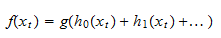whereis a decision tree.

### Train the boosted classifier

``````#Import the lightGBM package
import lightgbm as lgb

# Prepare dataset in LightGMB format
y_train = np.squeeze(y_train)
y_val = np.squeeze(y_val)
y_test = np.squeeze(y_test)
train_set = lgb.Dataset(x_train, y_train, silent=True)
valid_set = lgb.Dataset(x_val, y_val, silent=True)

# Set some algorithm parameters
params = {
'objective': 'binary',
'learning_rate': 0.1,
'metric': 'binary_logloss',
'min_data_in_leaf': 10,
'max_depth': 2,
}

# Train the model
boosted_tree = lgb.train(
params = params,
train_set = train_set,
valid_sets = valid_set,
num_boost_round = 10000,
early_stopping_rounds = 20,
verbose_eval = -1,
)

# Make predictions on the train, validation and test sets
y_train_pred = boosted_tree.predict(x_train)
y_val_pred = boosted_tree.predict(x_val)
y_test_pred = boosted_tree.predict(x_test)

# Print empirical risk on all sets
print('Log-loss on training set:')
print(log_loss(y_train, y_train_pred))
print('Log-loss on validation set:')
print(log_loss(y_val, y_val_pred))
print('Log-loss on test set:')
print(log_loss(y_test, y_test_pred))
print('')

# Print Accuracy on all sets
print('Accuracy on training set:')
print(accuracy_score(y_train, np.round(y_train_pred)))
print('Accuracy on validation set:')
print(accuracy_score(y_val, np.round(y_val_pred)))
print('Accuracy on test set:')
print(accuracy_score(y_test, np.round(y_test_pred)))``````

The output is:

``````Training until validation scores don't improve for 20 rounds
Early stopping, best iteration is:
	valid_0's binary_logloss: 0.290556
Log-loss on training set:
0.06289077335394931
Log-loss on validation set:
0.29055631956720585
Log-loss on test set:
0.3679290984742182

Accuracy on training set:
1.0
Accuracy on validation set:
0.87
Accuracy on test set:
0.86``````

Again, the performance of LightGBM is pretty impressive and much better than that of other models.